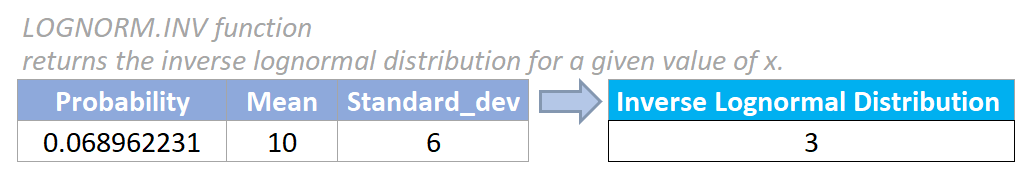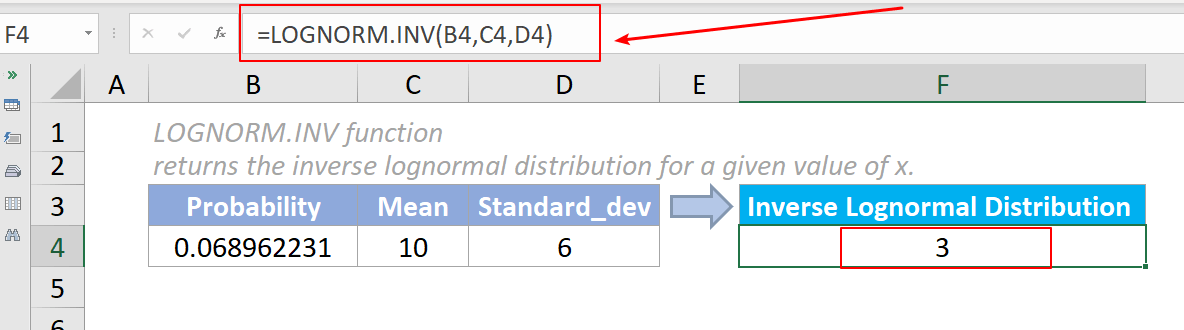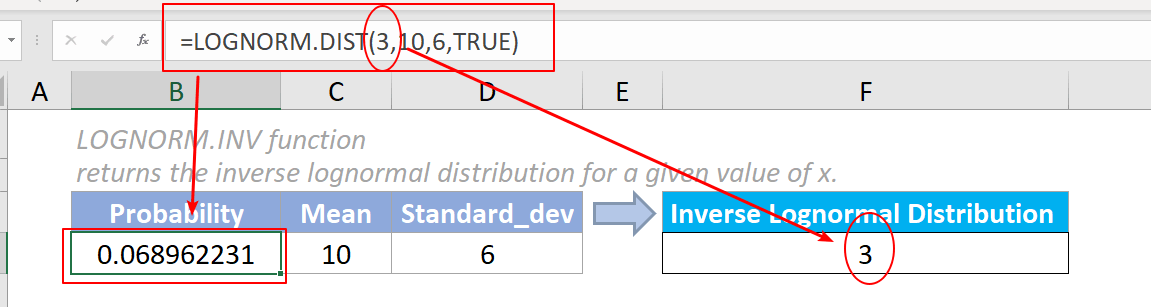## Excel LOGNORM.INV Function

The LOGNORM.INV function returns the inverse lognormal distribution for a given value of x. We can use the lognormal distribution to analyze data that has been logarithmically transformed.#### Syntax

=LOGNORM.INV(probability,mean,standard_dev)

#### Arguments

• Probability (required): The probability associated with the lognormal distribution.
• Mean (required): The arithmetic value of the mean of ln(x).
• Standard_dev (required): The value of the standard deviation of ln(x).

#### Return Value

The LOGNORM.INV function returns a numeric value.

#### Function notes

1. The LOGNORM.INV function was newly introduced In Excel 2010, so it is unavailable in earlier versions.
2. The #VALUE! error value occurs if any of the supplied arguments is non-numeric.
3. The #NUM! error value occurs if if any of the below situation occurs:
• The supplied probability is ≤ 0 or ≥ 1;
• The supplied standard_dev ≤ 0.
4. The LOGNORM.INV function returns the inverse of the cumulative lognormal distribution of x in the LOGNORM.DIST function. It means that if the value of possibility in LOGNORM.INV equals to result of LOGNORM.DIST(x, mean, standard_dev, cumulative), then the result of LOGNORM.INV(probability, mean, standard_dev) equals to the value of x in LOGNORM.DIST.

#### Example

As the below screenshot shows, there lists the values of probability, mean, and standard_dev parameters, to get the inverse of the cumulative lognormal distribution, please do as follows:

Please copy the formula below into cell F4, then press the Enter key to get the result.

=LOGNORM.INV(B4,C4,D4)Notes:

1. To show you the relationship between LOGNORM.INV and LOGNORM.DIST, the probability in the data above is the result of the LOGNORM.DIST function.You can clearly see that when probability = LOGNORM.DIST(x,...), then LOGNORM.INV(probability,...) = x.

2. We can also directly input values in the formula. The formula in cell F4 can be changed to:

=LOGNORM.INV(LOGNORM.DIST(3,10,6,TRUE),10,6)

#### Relative Functions:

• Excel EVEN Function
The EVEN function rounds numbers away from zero to the nearest even integer.

• Excel EXP Function
The EXP function returns the result of the constant e raised to the nth power.